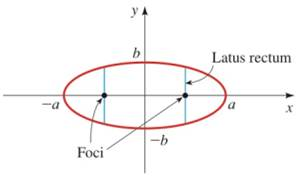Chapter 12.2, Problem 64E### Algebra and Trigonometry (MindTap ...

4th Edition
James Stewart + 2 others
ISBN: 9781305071742

#### Solutions

Chapter
Section### Algebra and Trigonometry (MindTap ...

4th Edition
James Stewart + 2 others
ISBN: 9781305071742
Textbook Problem

# How Wide Is an Ellipse at a Focus? A latus rectum for an ellipse is a line segment perpendicular to the major axis at a focus, with endpoints on the ellipse, as shown in the figure. Show that the length of a latus rectum is 2 b 2 / a for the ellipse x 2 a 2 + y 2 b 2 = 1 a > bTo determine

To show:

The length of a latus rectum is 2b2/a for the ellipse

x2a2+y2b2=1,a>b

Explanation

Approach:

A latus rectum for an ellipse is a line segment perpendicular to the major axis at a focus, with endpoints on the ellipse, as shown in the figure (1).

Figure (1)

Consider the equation of ellipse and figure (1),

x2a2+y2b2=1,a>b(1)

In equation (1),

b2=a2c2b>0

Solve equation (1) of the ellipse for y.

Calculation:

The equation of ellipse is,

x2a2+y2b2=1

From figure (1), the distance of x is equal to c.

Solve equation (1) of the ellipse for y

### Still sussing out bartleby?

Check out a sample textbook solution.

See a sample solution

#### The Solution to Your Study Problems

Bartleby provides explanations to thousands of textbook problems written by our experts, many with advanced degrees!

Get Started